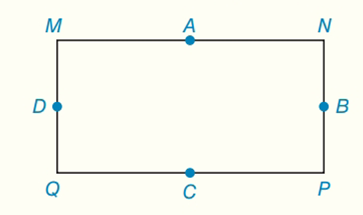Chapter 7.CT, Problem 16CT### Elementary Geometry for College St...

6th Edition
Daniel C. Alexander + 1 other
ISBN: 9781285195698

#### Solutions

Chapter
Section### Elementary Geometry for College St...

6th Edition
Daniel C. Alexander + 1 other
ISBN: 9781285195698
Textbook Problem
1 views

# For rectangle MNPQ, points A, B, C and D are the midpoints of the sides.a) What type of quadrilateral (not shown) is ABCD? _____________________b) Are the inscribed circle for ABCD and the circumscribed circle for MNPQ concentric circles? _____________________To determine

(a)

To find:

ABCD is what type of quadrilateral.

Explanation

Properties of rhombus:

1. All sides are congruent.

2. Diagonals are perpendicular bisectors of each other.

3. Each diagonal is the angle bisector of both the opposite angles.

4. Every rhombus is a parallelogram and a kite with all sides of equal length.

Calculation:

Given:

For rectangle MNPQ, points A, B, C and D are the midpoints of the sides.

Now we have to what type of quadrilateral (not shown) is ABCD...

To determine

(b)

To find:

The inscribed circle for ABCD and the circumscribed circle for MNPQ are concentric circles.

### Still sussing out bartleby?

Check out a sample textbook solution.

See a sample solution

#### The Solution to Your Study Problems

Bartleby provides explanations to thousands of textbook problems written by our experts, many with advanced degrees!

Get Started

#### Place the following set of n = 20 scores in a frequency distribution table.

Essentials of Statistics for The Behavioral Sciences (MindTap Course List)

#### In Exercises 516, evaluate the given quantity. log100,000

Finite Mathematics and Applied Calculus (MindTap Course List)

#### Write the number in the form a + bi. 41. ei/2

Single Variable Calculus: Early Transcendentals

#### In Exercises 69-74, rationalize the numerator. 69. 2x3

Applied Calculus for the Managerial, Life, and Social Sciences: A Brief Approach